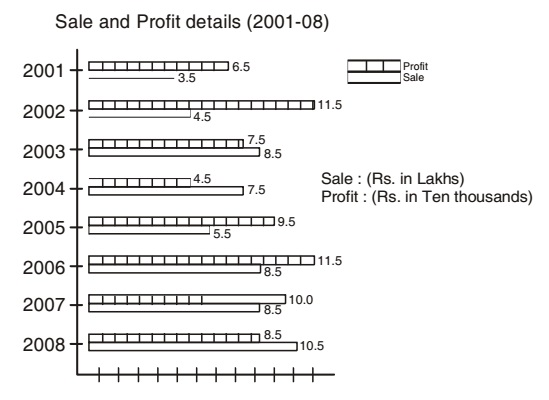Home » Data Interpretation » Bar graph » Question

#### Data Interpretation

Direction: The bar graph as shows below gives information about the sale and profit details of a departmental store during the years from 2001 - 2008. Study the graph carefully and answer the questions asked here under.1. During which of the following years percentage of profit earned by the store on the total sale was at the highest level?
1. 2001
2. 2002
3. 2003
4. 2005
##### Correct Option: B

Percentage of profit on the total in 2001 = Total profit in rupees x 100/ total sale in rupees.
Percentage of profit on the total in 2001 = 6.5 x 10000 x 100/3.5 x 100000 = 18.57
Percentage of profit on the total in 2002 = 11.5 x 10000 x 100/4.5 x 100000 = 25.56
Percentage of profit on the total in 2003 = 7.5 x 10000 x 100/8.5 x 100000 = 8.82
And Percentage of profit on the total in 2005 = 9.5 x 10000 x 100/ 8.5 x 100000 = 8.82
∴ Percentage of profit on the total sale in 2002 was the highest level.